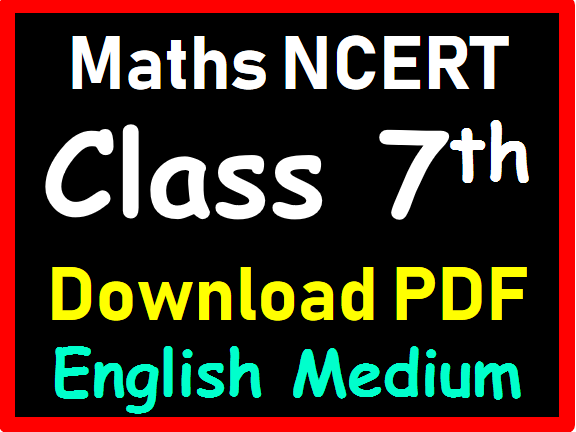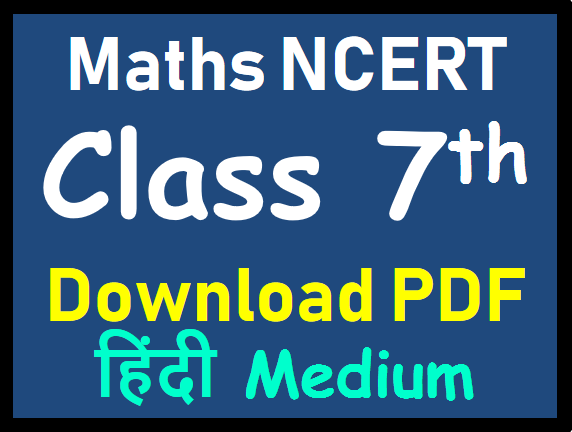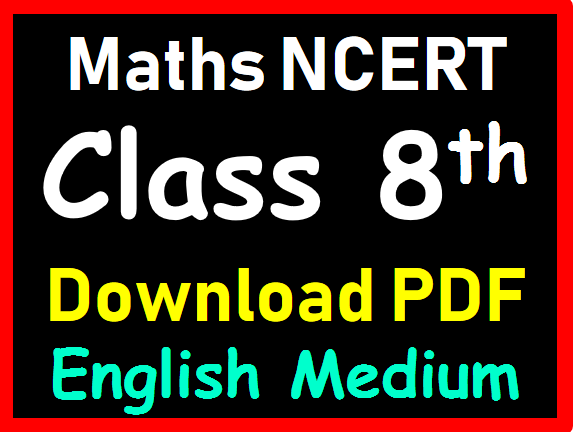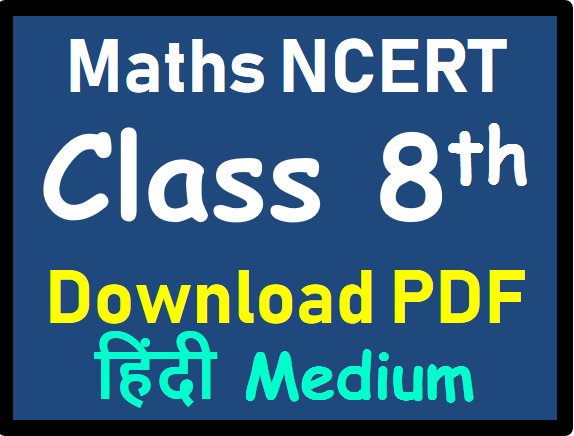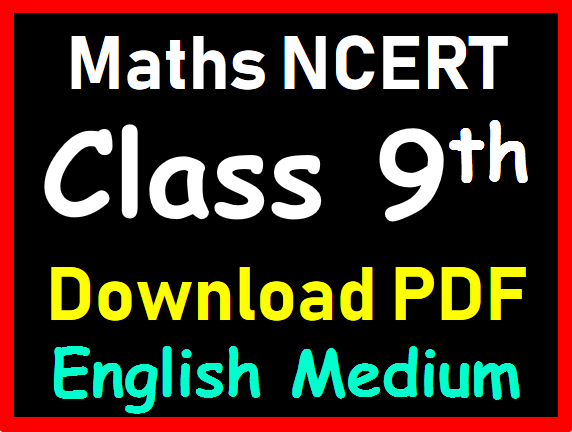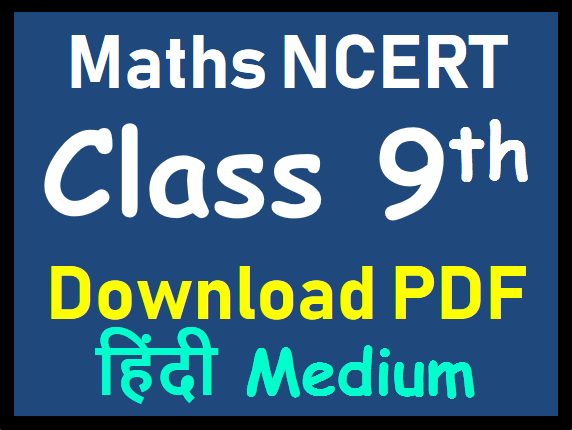# Importance of NCERT for SSC CGL Maths Preparation

NCERT books are considered to be the most important books if you are studying for board exams. The students must ensure that all the contents in the books are properly understood. The concepts in the books have been very carefully and nicely explained in self-explanatory manner which are easy to learn and understand.

NCERT is sufficient source to complete Maths portion of SSC CGL CHSL or any other SSC Exam. Every SSC Aspirant who is preparing for SSC CGL or CHSL Exam must refer NCERTs at least once to cover all the concepts of Advance Maths. Here you will ALL PDFs of Maths NCERTs.

There are some key benefits of studying NCERT books while preparing for SSC Exams and NCERT Exemplar Problems, for SSC Aspirants are stated below:
• Provide in-depth knowledge in easy language. …
• Sufficient Source, no need to buy any other material. …
• Clears all fundamental concepts. …
• Best for the SSC and Railway exams. …
• Offers a number of basic problems to practice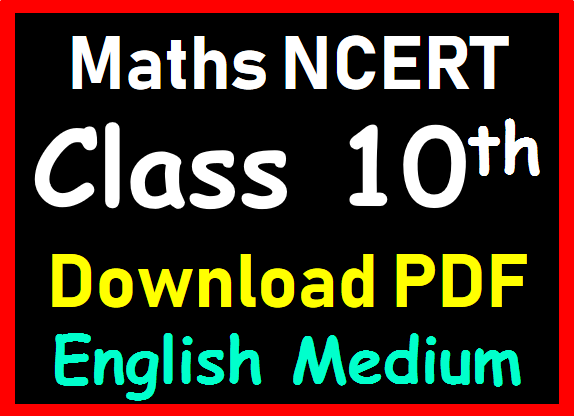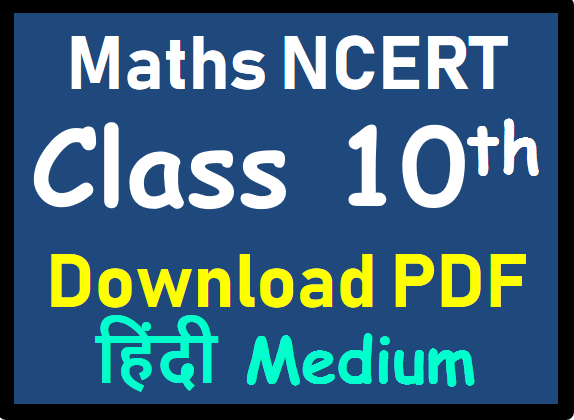## Class 10th Maths NCERT

COMPLETE SYLLABUS (Contents)

1. Real Numbers 1
1.1 Introduction 1
1.2 Euclid’s Division Lemma 2
1.3 The Fundamental Theorem of Arithmetic 7
1.4 Revisiting Irrational Numbers 11
1.5 Revisiting Rational Numbers and Their Decimal Expansions 15
1.6 Summary 18

2. Polynomials 20
2.1 Introduction 20
2.2 Geometrical Meaning of the Zeroes of a Polynomial 21
2.3 Relationship between Zeroes and Coefficients of a Polynomial 28
2.4 Division Algorithm for Polynomials 33
2.5 Summary 37

3. Pair of Linear Equations in Two Variables 38
3.1 Introduction 38
3.2 Pair of Linear Equations in Two Variables 39
3.3 Graphical Method of Solution of a Pair of Linear Equations 44
3.4 Algebraic Methods of Solving a Pair of Linear Equations 50
3.4.1 Substitution Method 50
3.4.2 Elimination Method 54
3.4.3 Cross-Multiplication Method 57
3.5 Equations Reducible to a Pair of Linear Equations in Two Variables 63
3.6 Summary 69

4.1 Introduction 70
2019-20
4.3 Solution of a Quadratic Equation by Factorisation 74
4.4 Solution of a Quadratic Equation by Completing the Square 76
4.5 Nature of Roots 88
4.6 Summary 91

5. Arithmetic Progressions 93
5.1 Introduction 93
5.2 Arithmetic Progressions 95
5.3 nth Term of an AP 100
5.4 Sum of First n Terms of an AP 107
5.5 Summary 116

6. Triangles 117
6.1 Introduction 117
6.2 Similar Figures 118
6.3 Similarity of Triangles 123
6.4 Criteria for Similarity of Triangles 129
6.5 Areas of Similar Triangles 141
6.6 Pythagoras Theorem 144
6.7 Summary 154

7. Coordinate Geometry 155
7.1 Introduction 155
7.2 Distance Formula 156
7.3 Section Formula 162
7.4 Area of a Triangle 168
7.5 Summary 172

8. Introduction to Trigonometry 173
8.1 Introduction 173
8.2 Trigonometric Ratios 174
8.3 Trigonometric Ratios of Some Specific Angles 181
8.4 Trigonometric Ratios of Complementary Angles 187
8.5 Trigonometric Identities 190
8.6 Summary 194

9. Some Applications of Trigonometry 195
9.1 Introduction 195
9.2 Heights and Distances 196
9.3 Summary 205

10. Circles 206
10.1 Introduction 206
10.2 Tangent to a Circle 207
10.3 Number of Tangents from a Point on a Circle 209
10.4 Summary 215

11. Constructions 216
11.1 Introduction 216
11.2 Division of a Line Segment 216
11.3 Construction of Tangents to a Circle 220
11.4 Summary 222

12. Areas Related to Circles 223
12.1 Introduction 223
12.2 Perimeter and Area of a Circle — A Review 224
12.3 Areas of Sector and Segment of a Circle 226
12.4 Areas of Combinations of Plane Figures 231
12.5 Summary 238

13. Surface Areas and Volumes 239
13.1 Introduction 239
13.2 Surface Area of a Combination of Solids 240
13.3 Volume of a Combination of Solids 245
13.4 Conversion of Solid from One Shape to Another 248
13.5 Frustum of a Cone 252
13.6 Summary 258

14. Statistics 260
14.1 Introduction 260
14.2 Mean of Grouped Data 260
14.3 Mode of Grouped Data 272
14.4 Median of Grouped Data 277
14.5 Graphical Representation of Cumulative Frequency Distribution 289
14.6 Summary 293

15. Probability 295
15.1 Introduction 295
15.2 Probability — A Theoretical Approach 296
15.3 Summary 312

# Maths NCERTs from Class 6th to 10th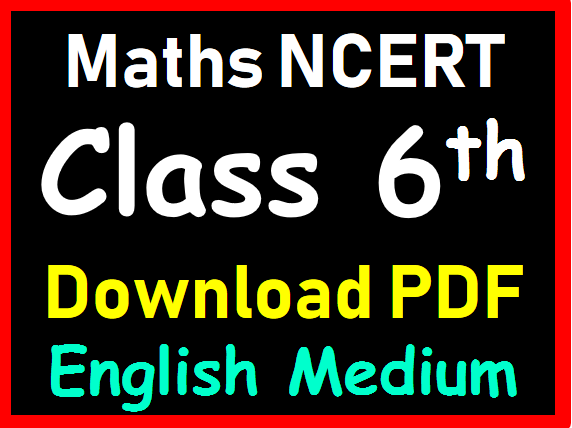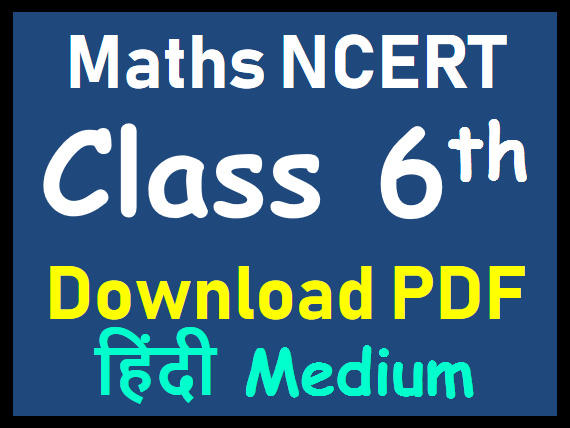##